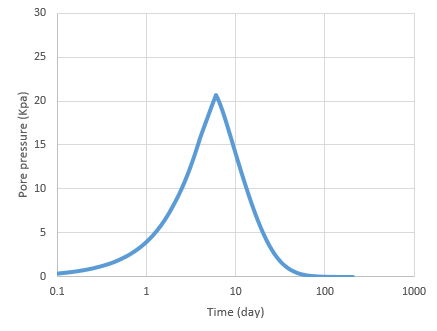پکیج آموزش پیشرفته آباکوس
پکیج جامع آباکوس ویژه رشته مکانیک
پکیج جامع آباکوس ژئوتکنیک و عمران

Sequential construction of an embankment on a clay layer Abaqus

This example been chosen from Example 4.7 Sam Helwany book

The embankment is constructed in three equal layers, each 0.6 m thick. Each embankment layer is constructed in a two-day period, during which consolidation of the clay layer takes place. The total construction time is six days

In this example, we use 5 steps , step 1 is Geostatic step ,The geostatic option make sure that equilibrium is satisfied within the clay layer

In step 2 we construct the first embankment layer ,In step 3 we construct the second embankment layer , In step 4 we construct the third (last) embankment layer and in Step 5 is a consolidation step with a duration of 200 days

Assume that the embankment material is linear elastic with Density of 1923 kg/m3, E = 478 kPa, Poisson ratio of 0.3, k = 0.1 m/s, and e0 = 1.5. Assume that the clay layer is elastoplastic, obeying the extended Cam clay model. The Cam clay model parameters for the clay layer are given in Table 4.5

We consider effective specific weight of each embankment layer with using body-force” option

In this example ,The groundwater table is coincident with the top surface of the clay layer

We intend to calculate the consolidation settlement under the center of the embankment

We intend to calculate the excess pore pressure in the middle of a clay layer under the center an embankmentCalculated excess pore pressure in the middle of a clay layer under the center of an embankment from AbaqusCalculated excess pore pressure in the middle of a clay layer under the center of an embankment from Sam Helwany book